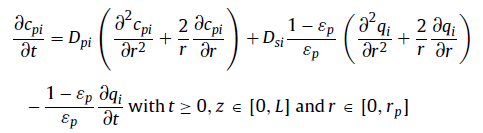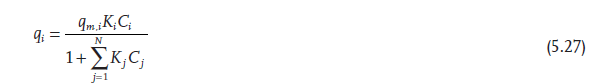# Adsorption processes in rapid equilibrium

Hi Heymann,

Sorry to disturb you again. I am very confused about the adsorption and have another three follow-up questions.

1. Multi-component Langmuir is used to describing the adsorption process. Based on this equation, if I set is_kinetic to be 1, then ka/kd and kd (or ka) should have effects on dq/dt. But I tried with the same keq but different kd values (e.g., ka=900, kd=1; ka=9000, kd=10; ka=90000, kd=100), the elution curves are the same. However, kd should have some effects from this equation.

2. Another problem is that if I set is_kinetic value to be 0, does this mean that the dq/dt is always equal to 0 and we can directly reduce this term dq/dt to be 0 in the mass balance of resin particles.3. In the book Protein Chromatography (Giorgio Carta and Alois Jungbauer), the following equation is used to describe the multi-component system. If we take the derivative of equation 5.27, the derivative term dqi/dt will be different from what I have seen in the first figure. If we use the derivative of 5.27, then dqi/dt will be only related to K (i.e, keq=ka/kd)Any suggestion would be much appreciated. Thanks in advance.
Best,
Chaoying

Dear Chaoying,

I have moved you questions to a new topic, as they are not strictly related to your original question and might be relevant for a broader audience.

1. Your k_a and k_d values are quite high. Try decreasing them by orders of magnitude and you should see the expected result. For large values, the adsorption process is not rate limiting and increasing them even further makes no difference. The results will be practically identical with what you get for IS_KINETIC=0.

2. This is an apparent paradox. Setting \frac{dq}{dt}=0 in the isotherm equation means that c and q are always in equilibrium. However, molecules of course still migrate between the mobile and stationary phases. Hence, the term with \frac{dq}{dt} needs to stay in the mass balance equation.

3. This actually follows from the previous point. You can use the mentioned trick if using IS_KINETIC=0 and wanting to know \frac{dq}{dt} for the mass balance. However, CADET solves the resulting differential-algebraic equations (DAE) in a different and numerically more efficient way.

I hope this helps.

Best regards
Eric

1 Like

Sorry for the late reply because I have been occupied with other stuff. Thanks so much for your reply, Eric. This is really helpful. I will try smaller values of ka and kd to see the difference.

Best,
Chaoying